## Polar Coordinates

### Learning Objectives

In this section, you will:

• Plot points using polar coordinates.
• Convert from polar coordinates to rectangular coordinates.
• Convert from rectangular coordinates to polar coordinates.
• Transform equations between polar and rectangular forms.
• Identify and graph polar equations by converting to rectangular equations.

Over 12 kilometers from port, a sailboat encounters rough weather and is blown off course by a 16-knot wind (see (Figure)). How can the sailor indicate his location to the Coast Guard? In this section, we will investigate a method of representing location that is different from a standard coordinate grid.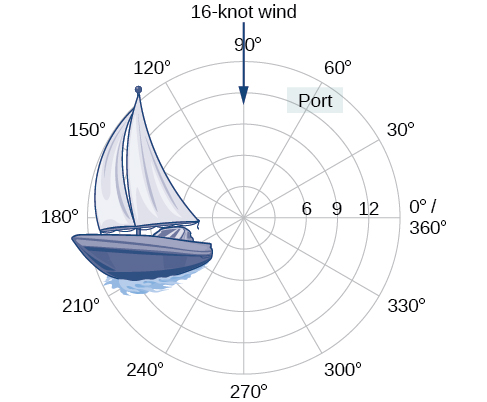Figure 1.

### Plotting Points Using Polar Coordinates

When we think about plotting points in the plane, we usually think of rectangular coordinates$\,\left(x,y\right)\,$in the Cartesian coordinate plane. However, there are other ways of writing a coordinate pair and other types of grid systems. In this section, we introduce to polar coordinates, which are points labeled$\,\left(r,\theta \right)\,$and plotted on a polar grid. The polar grid is represented as a series of concentric circles radiating out from the pole, or the origin of the coordinate plane.

The polar grid is scaled as the unit circle with the positive x-axis now viewed as the polar axis and the origin as the pole. The first coordinate$\,r\,$is the radius or length of the directed line segment from the pole. The angle$\,\theta ,$ measured in radians, indicates the direction of$\,r.\,$We move counterclockwise from the polar axis by an angle of$\,\theta ,$and measure a directed line segment the length of$\,r\,$in the direction of$\,\theta .\,$Even though we measure$\,\theta \,$first and then$\,r,$ the polar point is written with the r-coordinate first. For example, to plot the point$\,\left(2,\frac{\pi }{4}\right),$we would move$\,\frac{\pi }{4}\,$units in the counterclockwise direction and then a length of 2 from the pole. This point is plotted on the grid in (Figure).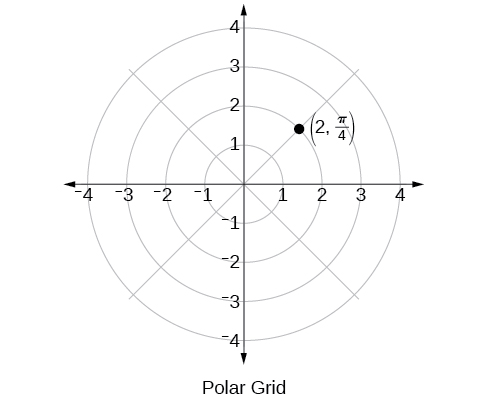Figure 2.

### Plotting a Point on the Polar Grid

Plot the point$\,\left(3,\frac{\pi }{2}\right)\,$on the polar grid.

### Try It

Plot the point$\,\left(2,\,\frac{\pi }{3}\right)\,$in the polar grid.

### Plotting a Point in the Polar Coordinate System with a Negative Component

Plot the point$\,\left(-2,\,\frac{\pi }{6}\right)\,$on the polar grid.

### Try It

Plot the points$\,\left(3,-\frac{\pi }{6}\right)$and$\,\left(2,\frac{9\pi }{4}\right)\,$on the same polar grid.

### Converting from Polar Coordinates to Rectangular Coordinates

When given a set of polar coordinates, we may need to convert them to rectangular coordinates. To do so, we can recall the relationships that exist among the variables$\,x,\,y,\,r,\,$and$\,\theta .$

$\begin{array}{l}\begin{array}{l}\\ \mathrm{cos}\,\theta =\frac{x}{r}\to x=r\mathrm{cos}\,\theta \end{array}\hfill \\ \mathrm{sin}\,\theta =\frac{y}{r}\to y=r\mathrm{sin}\,\theta \hfill \end{array}$

Dropping a perpendicular from the point in the plane to the x-axis forms a right triangle, as illustrated in (Figure). An easy way to remember the equations above is to think of$\,\mathrm{cos}\,\theta \,$as the adjacent side over the hypotenuse and$\,\mathrm{sin}\,\theta \,$as the opposite side over the hypotenuse.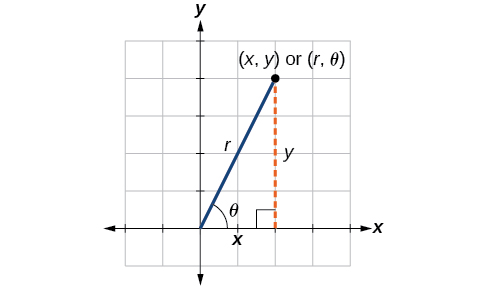Figure 5.

### Converting from Polar Coordinates to Rectangular Coordinates

To convert polar coordinates$\,\left(r,\,\theta \right)\,$to rectangular coordinates$\,\left(x,\,y\right),$ let

$\mathrm{cos}\,\theta =\frac{x}{r}\to x=r\mathrm{cos}\,\theta$
$\mathrm{sin}\,\theta =\frac{y}{r}\to y=r\mathrm{sin}\,\theta$

### How To

Given polar coordinates, convert to rectangular coordinates.

1. Given the polar coordinate$\,\left(r,\theta \right),$ write$\,x=r\mathrm{cos}\,\theta \,$and$\,y=r\mathrm{sin}\,\theta .$
2. Evaluate$\,\mathrm{cos}\,\theta \,$and$\,\mathrm{sin}\,\theta .$
3. Multiply$\,\mathrm{cos}\,\theta \,$by$\,r\,$to find the x-coordinate of the rectangular form.
4. Multiply$\,\mathrm{sin}\,\theta \,$by$\,r\,$to find the y-coordinate of the rectangular form.

### Writing Polar Coordinates as Rectangular Coordinates

Write the polar coordinates$\,\left(3,\frac{\pi }{2}\right)\,$as rectangular coordinates.

### Writing Polar Coordinates as Rectangular Coordinates

Write the polar coordinates$\,\left(-2,0\right)\,$as rectangular coordinates.

### Try It

Write the polar coordinates$\,\left(-1,\frac{2\pi }{3}\right)\,$as rectangular coordinates.

### Converting from Rectangular Coordinates to Polar Coordinates

To convert rectangular coordinates to polar coordinates, we will use two other familiar relationships. With this conversion, however, we need to be aware that a set of rectangular coordinates will yield more than one polar point.

### Converting from Rectangular Coordinates to Polar Coordinates

Converting from rectangular coordinates to polar coordinates requires the use of one or more of the relationships illustrated in (Figure).

$\begin{array}{l}\mathrm{cos}\,\theta =\frac{x}{r}\,\,\text{ or}\,\,\,x=r\mathrm{cos}\,\theta \hfill \\ \mathrm{sin}\,\theta =\frac{y}{r}\,\,\text{ or}\,\,\,y=r\mathrm{sin}\,\theta \hfill \\ \,\,\,\,\,\,{r}^{2}={x}^{2}+{y}^{2}\hfill \\ \mathrm{tan}\,\theta =\frac{y}{x}\,\hfill \end{array}$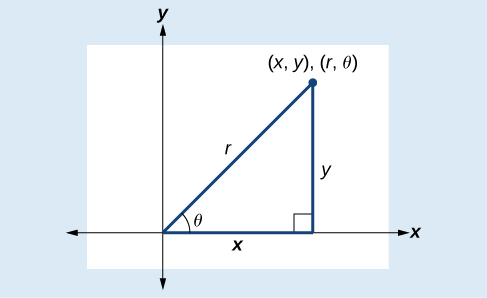Figure 8.

### Writing Rectangular Coordinates as Polar Coordinates

Convert the rectangular coordinates$\,\left(3,3\right)\,$to polar coordinates.

#### Analysis

There are other sets of polar coordinates that will be the same as our first solution. For example, the points$\,\left(-3\sqrt{2},\,\frac{5\pi }{4}\right)\,$and$\,\left(3\sqrt{2},-\frac{7\pi }{4}\right)\,$will coincide with the original solution of$\,\left(3\sqrt{2},\,\frac{\pi }{4}\right).\,$The point$\,\left(-3\sqrt{2},\,\frac{5\pi }{4}\right)\,$indicates a move further counterclockwise by$\,\pi ,\,$which is directly opposite$\,\frac{\pi }{4}.\,$The radius is expressed as$\,-3\sqrt{2}.\,$However, the angle$\,\frac{5\pi }{4}\,$is located in the third quadrant and, as$\,r\,$is negative, we extend the directed line segment in the opposite direction, into the first quadrant. This is the same point as$\,\left(3\sqrt{2},\,\,\frac{\pi }{4}\right).\,$The point$\,\left(3\sqrt{2},\,-\frac{7\pi }{4}\right)\,$is a move further clockwise by$\,-\frac{7\pi }{4},\,$from$\,\frac{\pi }{4}.\,$The radius,$\,3\sqrt{2},\,$is the same.

### Transforming Equations between Polar and Rectangular Forms

We can now convert coordinates between polar and rectangular form. Converting equations can be more difficult, but it can be beneficial to be able to convert between the two forms. Since there are a number of polar equations that cannot be expressed clearly in Cartesian form, and vice versa, we can use the same procedures we used to convert points between the coordinate systems. We can then use a graphing calculator to graph either the rectangular form or the polar form of the equation.

### How To

Given an equation in polar form, graph it using a graphing calculator.

1. Change the MODE to POL, representing polar form.
2. Press the Y= button to bring up a screen allowing the input of six equations:$\,{r}_{1},\,\,{r}_{2},\,\,.\,\,.\,\,.\,\,,\,\,{r}_{6}.$
3. Enter the polar equation, set equal to$\,r.$
4. Press GRAPH.

### Writing a Cartesian Equation in Polar Form

Write the Cartesian equation$\,{x}^{2}+{y}^{2}=9\,$in polar form.

### Rewriting a Cartesian Equation as a Polar Equation

Rewrite the Cartesian equation$\,{x}^{2}+{y}^{2}=6y\,$as a polar equation.

### Rewriting a Cartesian Equation in Polar Form

Rewrite the Cartesian equation$\,y=3x+2\,$as a polar equation.

### Try It

Rewrite the Cartesian equation$\,{y}^{2}=3-{x}^{2}\,$in polar form.

### Identify and Graph Polar Equations by Converting to Rectangular Equations

We have learned how to convert rectangular coordinates to polar coordinates, and we have seen that the points are indeed the same. We have also transformed polar equations to rectangular equations and vice versa. Now we will demonstrate that their graphs, while drawn on different grids, are identical.

### Graphing a Polar Equation by Converting to a Rectangular Equation

Covert the polar equation$\,r=2\mathrm{sec}\,\theta \,$ to a rectangular equation, and draw its corresponding graph.

### Rewriting a Polar Equation in Cartesian Form

Rewrite the polar equation$\,r=\frac{3}{1-2\mathrm{cos}\,\theta }\,$as a Cartesian equation.

#### Analysis

In this example, the right side of the equation can be expanded and the equation simplified further, as shown above. However, the equation cannot be written as a single function in Cartesian form. We may wish to write the rectangular equation in the hyperbola’s standard form. To do this, we can start with the initial equation.

$\begin{array}{ll}\,\text{ }{x}^{2}+{y}^{2}={\left(3+2x\right)}^{2}\hfill & \hfill \\ \,\,\text{ }{x}^{2}+{y}^{2}-{\left(3+2x\right)}^{2}=0\hfill & \hfill \\ {x}^{2}+{y}^{2}-\left(9+12x+4{x}^{2}\right)=0\hfill & \hfill \\ \,\text{ }{x}^{2}+{y}^{2}-9-12x-4{x}^{2}=0\hfill & \hfill \\ \,\text{ }-3{x}^{2}-12x+{y}^{2}=9\hfill & \text{Multiply through by }-1.\hfill \\ \,\,\text{ }\,3{x}^{2}+12x-{y}^{2}=-9\hfill & \hfill \\ \,\,\text{ }\,3\left({x}^{2}+4x+\,\,\,\,\,\,\,\,\,\right)-{y}^{2}=-9\hfill & \text{Organize terms to complete the square for}\,x.\hfill \\ \,\,\text{ }\,3\left({x}^{2}+4x+4\right)-{y}^{2}=-9+12\hfill & \hfill \\ \text{ }3{\left(x+2\right)}^{2}-{y}^{2}=3\hfill & \hfill \\ \,\text{ }{\left(x+2\right)}^{2}-\frac{{y}^{2}}{3}=1\hfill & \hfill \end{array}$

### Try It

Rewrite the polar equation$\,r=2\mathrm{sin}\,\theta \,$ in Cartesian form.

### Rewriting a Polar Equation in Cartesian Form

Rewrite the polar equation$\,r=\mathrm{sin}\left(2\theta \right)\,$in Cartesian form.

Access these online resources for additional instruction and practice with polar coordinates.

### Key Equations

 Conversion formulas $\begin{array}{ll}\hfill & \mathrm{cos}\,\theta =\frac{x}{r}\to x=r\mathrm{cos}\,\theta \hfill \\ \hfill & \mathrm{sin}\,\theta =\frac{y}{r}\to y=r\mathrm{sin}\,\theta \hfill \\ \hfill & \,\,\,\,\,\,\,{r}^{2}={x}^{2}+{y}^{2}\hfill \\ \hfill & \mathrm{tan}\,\theta =\frac{y}{x}\hfill \end{array}$

### Key Concepts

• The polar grid is represented as a series of concentric circles radiating out from the pole, or origin.
• To plot a point in the form$\,\left(r,\theta \right),\,\theta >0,\,$move in a counterclockwise direction from the polar axis by an angle of $\,\theta ,\,$and then extend a directed line segment from the pole the length of $\,r\,$ in the direction of $\,\theta .\,$If$\,\theta \,$is negative, move in a clockwise direction, and extend a directed line segment the length of $\,r\,$ in the direction of$\,\theta .$ See (Figure).
• If$\,r\,$is negative, extend the directed line segment in the opposite direction of$\,\theta .\,$See (Figure).
• To convert from polar coordinates to rectangular coordinates, use the formulas$\,x=r\mathrm{cos}\,\theta \,$and$\,y=r\mathrm{sin}\,\theta .\,$See (Figure) and (Figure).
• To convert from rectangular coordinates to polar coordinates, use one or more of the formulas:$\,\mathrm{cos}\,\theta =\frac{x}{r},\mathrm{sin}\,\theta =\frac{y}{r},\mathrm{tan}\,\theta =\frac{y}{x},\,$and$\,r=\sqrt{{x}^{2}+{y}^{2}}.\,$See (Figure).
• Transforming equations between polar and rectangular forms means making the appropriate substitutions based on the available formulas, together with algebraic manipulations. See (Figure), (Figure), and (Figure).
• Using the appropriate substitutions makes it possible to rewrite a polar equation as a rectangular equation, and then graph it in the rectangular plane. See (Figure), (Figure), and (Figure).

### Section Exercises

#### Verbal

How are polar coordinates different from rectangular coordinates?

How are the polar axes different from the x– and y-axes of the Cartesian plane?

Explain how polar coordinates are graphed.

How are the points$\,\left(3,\frac{\pi }{2}\right)\,$and$\,\left(-3,\frac{\pi }{2}\right)\,$related?

Explain why the points$\,\left(-3,\frac{\pi }{2}\right)\,$and$\,\left(3,-\frac{\pi }{2}\right)\,$are the same.

#### Algebraic

For the following exercises, convert the given polar coordinates to Cartesian coordinates with$\,r>0\,$and$\,0\le \theta \le 2\pi .\,$Remember to consider the quadrant in which the given point is located when determining$\,\theta \,$for the point.

$\left(7,\frac{7\pi }{6}\right)$

$\left(5,\pi \right)$

$\left(6,-\frac{\pi }{4}\right)$

$\left(-3,\frac{\pi }{6}\right)$

$\left(4,\frac{7\pi }{4}\right)$

For the following exercises, convert the given Cartesian coordinates to polar coordinates with$\,r>0,\,\,0\le \theta <2\pi .\,$Remember to consider the quadrant in which the given point is located.

$\left(4,2\right)$

$\left(-4,6\right)$

$\left(3,-5\right)$

$\left(-10,-13\right)$

$\left(8,8\right)$

For the following exercises, convert the given Cartesian equation to a polar equation.

$x=3$

$y=4$

$y=4{x}^{2}$

$y=2{x}^{4}$

${x}^{2}+{y}^{2}=4y$

${x}^{2}+{y}^{2}=3x$

${x}^{2}-{y}^{2}=x$

${x}^{2}-{y}^{2}=3y$

${x}^{2}+{y}^{2}=9$

${x}^{2}=9y$

${y}^{2}=9x$

$9xy=1$

For the following exercises, convert the given polar equation to a Cartesian equation. Write in the standard form of a conic if possible, and identify the conic section represented.

$r=3\mathrm{sin}\,\theta$

$r=4\mathrm{cos}\,\theta$

$r=\frac{4}{\mathrm{sin}\,\theta +7\mathrm{cos}\,\theta }$

$r=\frac{6}{\mathrm{cos}\,\theta +3\mathrm{sin}\,\theta }$

$r=2\mathrm{sec}\,\theta$

$r=3\mathrm{csc}\,\theta$

$r=\sqrt{r\mathrm{cos}\,\theta +2}$

${r}^{2}=4\mathrm{sec}\,\theta \,\mathrm{csc}\,\theta$

$r=4$

${r}^{2}=4$

$r=\frac{1}{4\mathrm{cos}\,\theta -3\mathrm{sin}\,\theta }$

$r=\frac{3}{\mathrm{cos}\,\theta -5\mathrm{sin}\,\theta }$

#### Graphical

For the following exercises, find the polar coordinates of the point.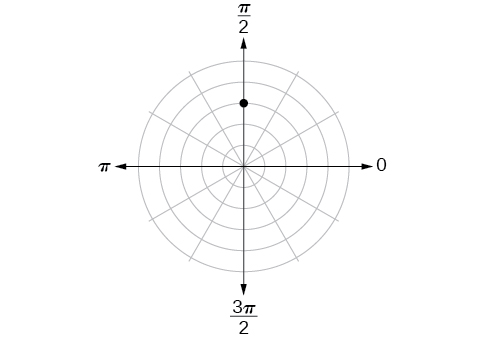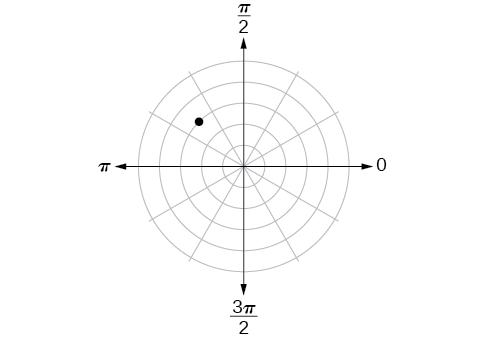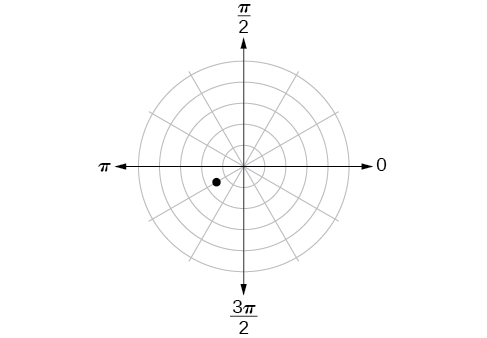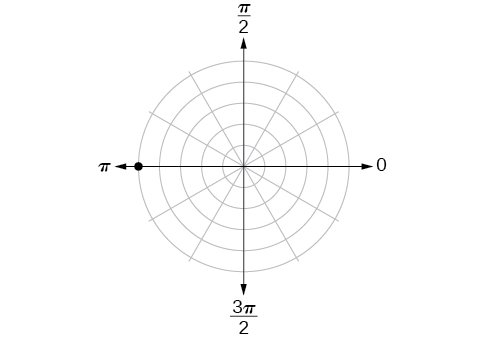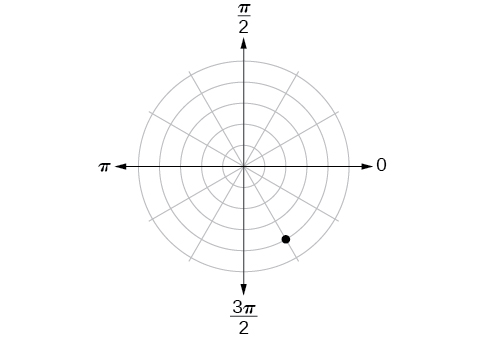For the following exercises, plot the points.

$\left(-2,\frac{\pi }{3}\right)$

$\left(-1,-\frac{\pi }{2}\right)$

$\left(3.5,\frac{7\pi }{4}\right)$

$\left(-4,\frac{\pi }{3}\right)$

$\left(5,\frac{\pi }{2}\right)$

$\left(4,\frac{-5\pi }{4}\right)$

$\left(3,\frac{5\pi }{6}\right)$

$\left(-1.5,\frac{7\pi }{6}\right)$

$\left(-2,\frac{\pi }{4}\right)$

$\left(1,\frac{3\pi }{2}\right)$

For the following exercises, convert the equation from rectangular to polar form and graph on the polar axis.

$5x-y=6$

$2x+7y=-3$

${x}^{2}+{\left(y-1\right)}^{2}=1$

${\left(x+2\right)}^{2}+{\left(y+3\right)}^{2}=13$

$x=2$

${x}^{2}+{y}^{2}=5y$

${x}^{2}+{y}^{2}=3x$

For the following exercises, convert the equation from polar to rectangular form and graph on the rectangular plane.

$r=6$

$r=-4$

$\theta =-\frac{2\pi }{3}$
$\theta =\frac{\pi }{4}$

$r=\mathrm{sec}\,\theta$

$r=-10\mathrm{sin}\,\theta$

$r=3\mathrm{cos}\,\theta$

#### Technology

Use a graphing calculator to find the rectangular coordinates of$\,\left(2,-\frac{\pi }{5}\right).\,$Round to the nearest thousandth.

Use a graphing calculator to find the rectangular coordinates of$\,\left(-3,\frac{3\pi }{7}\right).\,$Round to the nearest thousandth.

Use a graphing calculator to find the polar coordinates of$\,\left(-7,8\right)\,$in degrees. Round to the nearest thousandth.

Use a graphing calculator to find the polar coordinates of$\,\left(3,-4\right)\,$in degrees. Round to the nearest hundredth.

Use a graphing calculator to find the polar coordinates of$\,\left(-2,0\right)\,$in radians. Round to the nearest hundredth.

#### Extensions

Describe the graph of$\,r=a\mathrm{sec}\,\theta ;a>0.$

Describe the graph of$\,r=a\mathrm{sec}\,\theta ;a<0.$

Describe the graph of$\,r=a\mathrm{csc}\,\theta ;a>0.$

Describe the graph of$\,r=a\mathrm{csc}\,\theta ;a<0.$

What polar equations will give an oblique line?

For the following exercise, graph the polar inequality.

$r<4$

$0\le \theta \le \frac{\pi }{4}$

$\theta =\frac{\pi }{4},\,r\,\ge \,2$

$\theta =\frac{\pi }{4},\,r\,\ge -3$

$0\le \theta \le \frac{\pi }{3},\,r\,<\,2$

$\frac{-\pi }{6}<\theta \le \frac{\pi }{3},-3<r\,<\,2$

### Glossary

polar axis
on the polar grid, the equivalent of the positive x-axis on the rectangular grid
polar coordinates
on the polar grid, the coordinates of a point labeled$\,\left(r,\theta \right),\,$where$\,\theta \,$indicates the angle of rotation from the polar axis and$\,r\,$represents the radius, or the distance of the point from the pole in the direction of$\,\theta$
pole
the origin of the polar grid